##Pascal's theorem for triangles

Consider a triangle ABC inscribed in a conic. Then the intersection points of pairs of lines consisting by a side (f.e. BC) and the tangent to the opposite vertex (f.e. A) intersect at three points A*, B*, C* which are on the same line e. Line e coincides with the trilinear polar of point E with respect to triangle ABC, E being the pole of e w.r. to the conic.
Triangle A''B''C'' formed by the tangents of the conic at the vertices of ABC has for vertices the harmonic associates of E with respect to ABC. The conic is inscribed in this triangle.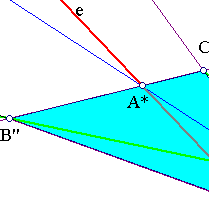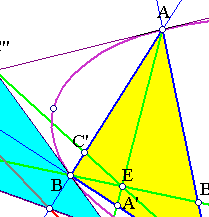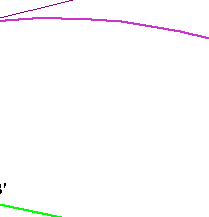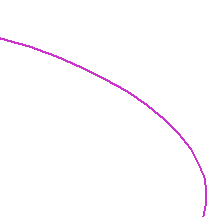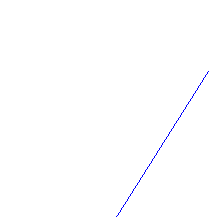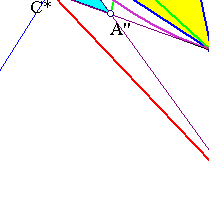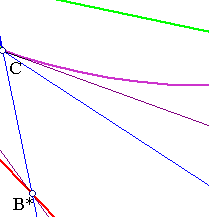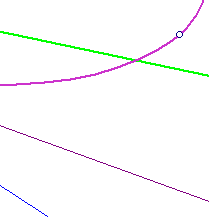Line e results by applying the theorem of Pascal on the triangle. Consider then one of these intersection points, C* say, on line e and take its harmonic conjugate C' on line ABC*. By the fundamental construction of the tripole, line CC', as well as the corresponding lines AA', BB' pass through a point E, which is the tripole (see Tripole.html ) of line e. By its construction CC' is the polar of C*. It follows that E is the pole of line e w.r. to the conic.
Given triangle ABC and a point E in its plane, the previous considerations show that there is a unique conic corresponding to the above figure. I mean that E defines the corresponding trilinear polar e, points A*, B*, C* and three lines A*A, B*B, C*C required to be tangent to the conic at A, B, C respectively.
The figure shows also, for three lines through the vertices of the triangle, the necessary condition to be the tangents to some circumconic of the triangle. Their intersection points with the corresponding opposite sides must be collinear (like A*, B*, C*). This is also sufficient, since then the line carrying A*,B*,C* determines the corresponding E and this the wanted conic (see AllConicsCircumscribedTria.html ).

There is a distinguished circumconic of particular importance. The outer Steiner ellipse, for which the tangents at the vertices are parallel to the opposite sides. E coincides with the centroid of ABC and the ellipse is the image by F of the circumcircle of A*B*C*. See the file ProjectivityFixingVertices.html and the references given below for a further discussion of the subject.

AllConicsCircumscribedTria.html
CrossRatio.html
CrossRatio0.html
CrossRatioLines.html
GoodParametrization.html
Harmonic.html
Harmonic_Bundle.html
HomographicRelation.html
HomographicRelationExample.html
Pascal.html